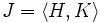# Join of two 3-subnormal subgroups may be proper and contranormal

## Statement

It is possible to have a group$G$ and two 3-subnormal subgroup (?)s$H$ and$K$ of$G$ such that the join$\langle H, K \rangle$ is not a Subnormal subgroup (?) of$G$. In fact, it can happen that the join is a proper Contranormal subgroup (?).

## Proof

### Construction of the counterexample

The construction involves the following steps:

• Let$S$ be the set of all subsets$X$ of$\mathbb{Z}$ such that there exists integers$l(X) \le L(X)$ such that$X$ contains all integers less than$l(X)$ and no integer greater than$L(X)$.
• Let$A$ be an elementary abelian$2$-group with basis (as a vector space over the field of two elements) given by$a_X$, where$X$ ranges over$S$.
• Let$B$ be an elementary abelian$2$-group with basis (as a vector space over the field of two elements) given by$b_X$, where$X$ ranges over$S$. (Note that$A$ and$B$ are isomorphic).
• Let$M$ be the direct product of$A$ and$B$.
• For every$n \in \mathbb{Z}$, define$a_{X*n} = a_{X \cup \{ n \}}$ if$n \notin X$, and$0$ if$n \in X$. Analogously, define$b_{X * n}$. Now define, for$n \in \mathbb{Z}$:
• Automorphisms$u_n:M \to M$ given on the basis by$u_n(a_X,b_Y) = (a_X, b_{X*n} + b_Y)$.
• Automorphisms$v_n:M \to M$ given on the basis by$v_n(a_X,b_Y) = (a_{Y*n} + a_X, b_Y)$.
• Let$H$ be the subgroup of$\operatorname{Aut}(M)$ generated by the$u_n$ and$K$ be the subgroup of$\operatorname{Aut}(M)$ generated by the$v_n$.
• Define$J = \langle H, K \rangle$, again as a subgroup of$\operatorname{Aut}(M)$.
• Define$G$ as the external semidirect product$M \rtimes J$, with the action of$J$ the usual action by automorphisms.

Then, both$H$ and$K$ are$3$-subnormal subgroups of$G$, but$J = \langle H, K \rangle$ is not a subnormal subgroup of$G$.

### Preliminary computations

Claim:$[H,A] = B$ and$[K,B] = A$.

### Proof that$H$ and$K$ are both$3$-subnormal

We prove that$H$ is$3$-subnormal in three steps:

• The normal closure of$H$ in$G$ is$H^KM$: PLACEHOLDER FOR INFORMATION TO BE FILLED IN: [SHOW MORE]
• The normal closure of$H$ in$H^KM$ is$HB$: PLACEHOLDER FOR INFORMATION TO BE FILLED IN: [SHOW MORE]
•$H$ is normal in$\langle H, B \rangle$: In fact,$\langle H, B \rangle$ is an internal direct product of$H$ and$B$.

### Proof that$J$ is proper and contranormal

The normal closure of$J$ in$G$ contains both$H^A = H[H,A] = HB$ and$K^B = K[K,B] = KA$. Thus, the normal closure of$J$ in$G$ contains$H,K,A,B$, and hence must be the whole group$G$.

That$J$ is proper follows because it is the non-normal part of a semidirect product with a nontrivial group$M$.